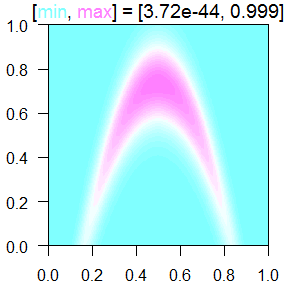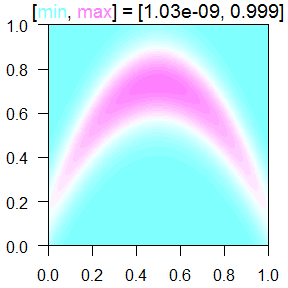An Introduction to TestFunctions

2017-05-09

This is an introduction to the R package TestFunctions. It is available on CRAN and is maintained through Github.

Test functions are used whenever one needs to evaluate an algorithm. For example, an optimization algorithm should be tested on many different functions to make sure that it works and is robust. Thus many optimization test functions are very tricky, such as those with many local minima meant to make the global minimum harder to find.

How do I use this package?

Each of the test functions is called like any other function. The first argument, x, should be a vector representing one point or a matrix that has points in its rows. This can cause problems if you are using a 1-dimensional function and pass in a vector of values. Instead you should pass them in as a matrix with a single column, or vectorize the function.

The code below shows how the branin function can be used, taking in either a vector or a matrix.

set.seed(0)
library(TestFunctions)
branin(runif(2))
##  9.476405
branin(matrix(runif(20), ncol=2))
##    24.119600  71.180268  18.374071   9.839029  36.607437  72.884496
##   196.302169  25.185022  13.059216  27.129463

A contour of the banana function is shown below.

ContourFunctions::cf(banana)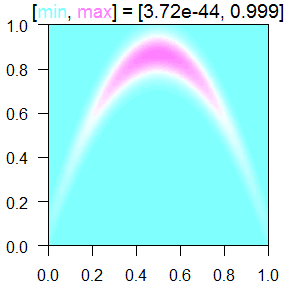General function information

The functions are all designed to be run by default in the $$[0,1]^D$$ unit cube. If you want to run the function on the original input values, you can set scale_it=FALSE.

Independent Gaussian noise can be added to most functions by passing the standard deviation of the noise as the noise parameter. The plots below show the original function, then what data from the function with noise looks like.

tf1 <- function(xx) powsin(x=matrix(xx,ncol=1),  noise=0)
curve(tf1, main="Function without noise")

x1 <- runif(1e2)
y1 <- powsin(x=matrix(x1,ncol=1),  noise=.1)
plot(x1,y1, col=2, pch=19, cex=.3, main="Data with noise")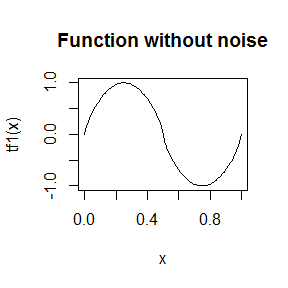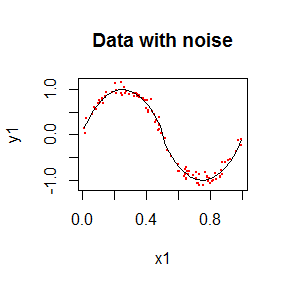Random wave functions

The function RFF_get will return a random wave function with any given number of dimensions. The function is created by combining many different one dimensional waves passing through the input area with various directions, magnitude, and offset. The default is composed of sine waves, but this can be changed to block or v waves.

Below is an example of a one dimensional wave.

tf <- RFF_get(D=1)
curve(tf)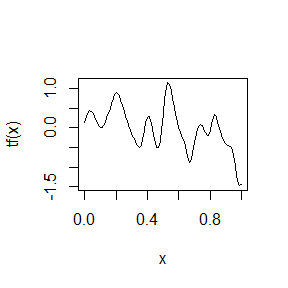Below is an example of a random wave in two dimensions.

ContourFunctions::cf(RFF_get(D=2))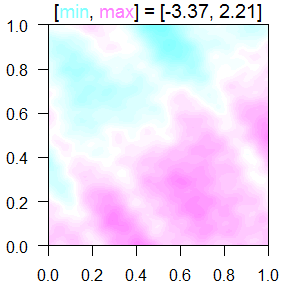Function enhancers

There are some functions that modify other functions.

• add_null_dims adds extra dimensions that do not affect the function output.

• add_zoom lets you zoom in on part of a function. Below are two examples of zooming in on the banana function.

ContourFunctions::cf(banana)